# Compositional Visual Generation and Inference with Energy Based Models

## Abstract

A vital aspect of human intelligence is the ability to compose increasingly complex concepts out of simpler ideas, enabling both rapid learning and adaptation of knowledge. In this paper we show that energy-based models can exhibit this ability by directly combining probability distributions. Samples from the combined distribution correspond to compositions of concepts. For example, given a distribution for smiling faces, and another for male faces, we can combine them to generate smiling male faces. This allows us to generate natural images that simultaneously satisfy conjunctions, disjunctions, and negations of concepts. We evaluate compositional generation abilities of our model on the CelebA dataset of natural faces and synthetic 3D scene images. We also demonstrate other unique advantages of our model, such as the ability to continually learn and incorporate new concepts, or infer compositions of concept properties underlying an image.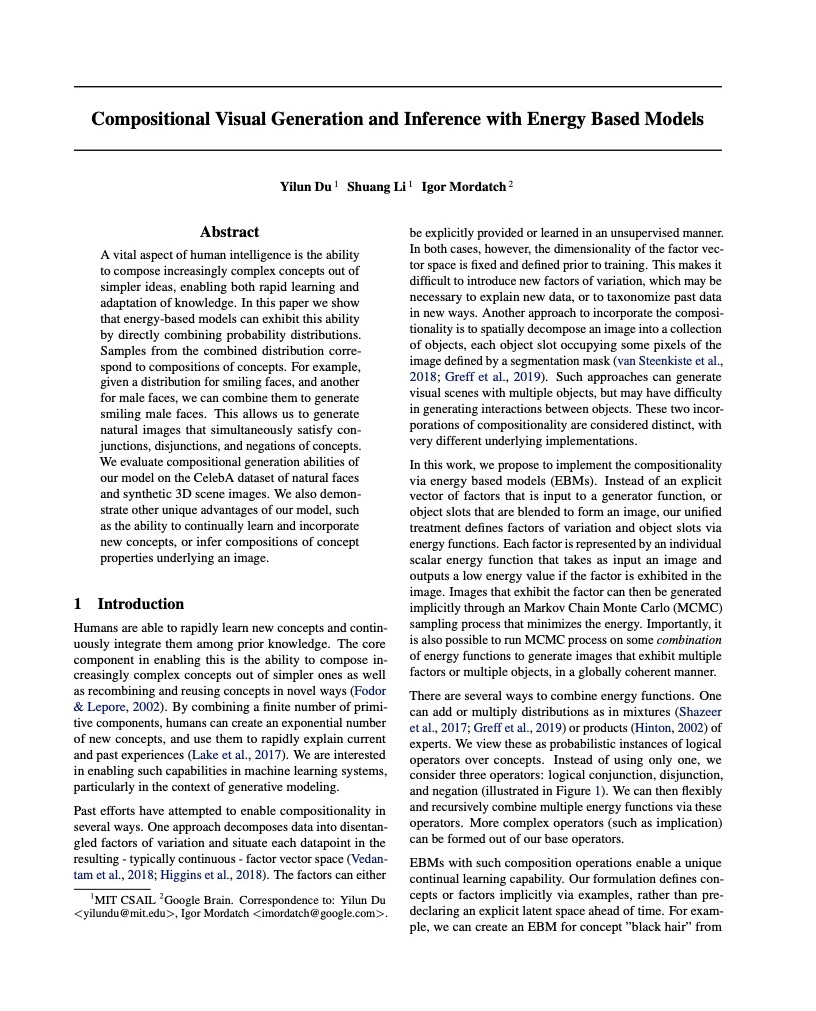## Paper

Neurips 2020 (Spotlight).

## Citation

Yilun Du, Shuang Li, Igor Mordatch. "Compositional Visual Generation and Inference with Energy Based Models", NeurIPS 2020 (spotlight). Bibtex

## Method

Energy based models (EBMs) represent a distribution over data by defining an energy $$E_\theta(x)$$ so that the likelihood of the data is proportional to $$\propto e^{-E_\theta(x)}$$. We can generate data from an EBM by implicit sampling through Langevin dynamics , where samples are sequentially refined, following the procedure $\tilde{\mathbb{x}}^k = \tilde{\mathbb{x}}^{k-1} - \frac{\lambda}{2} \nabla_\mathbb{x} E_\theta (\tilde{\mathbb{x}}^{k-1}) + \omega^k, \; \omega^k \sim \mathcal{N}(0,\lambda),$ By defining generation through such a manner, we can compose generation across different EBMs learned on attributes of position, size, color, gender, hair style, and age, through the symbolic operators of conjunction, disjunction, and negation. In particular, we consider a set of independently trained EBMs, $$E(\tilde{x}|c_1), E(\tilde{x}|c_2), \ldots, E(\tilde{x}|c_n)$$, which are learned conditional distributions on underlying latent codes $$c_i$$. Latent codes we consider include position, size, color, gender, hair style, and age, which we also refer to as concepts.

### Conjunction

In concept conjunction, given separate independent concepts (such as a particular gender, hair style, or facial expression), we wish to construct an generation with the specified gender, hair style, and facial expression -- the combination of each concept. The likelihood of such an generation given a set of specific concepts is equal to the product of the likelihood of each individual concept $p(x|c_1 \; \text{and} \; c_2, \ldots, \; \text{and} \; c_i) = \prod_i p(x|c_i) \propto e^{-\sum_i E(x|c_i)}.$ Through our implicit sampling procedure, we can generate samples using $\tilde{\mathbb{x}}^k = \tilde{\mathbb{x}}^{k-1} - \frac{\lambda}{2} \nabla_\mathbb{x} \sum_i E_\theta (\tilde{\mathbb{x}}^{k-1}|c_i) + \omega^k.$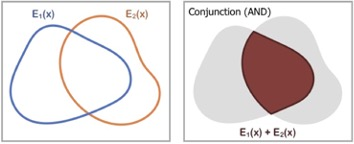### Disjunction

In concept disjunction, given separate concepts such as the colors red and blue, we wish to construct an output that is either red or blue. We wish to construct a new distribution that has probability mass when any chosen concept is true. A natural choice of such a distribution is the sum of the likelihood of each concept: $p(x|c_1 \; \text{or} \; c_2, \ldots \; \text{or} \; c_i) \propto \sum_i p(x|c_i) / Z(c_i).$ where $$Z(c_i)$$ denotes partition function for the chosen concept. Through our implicit sampling procedure, by assuming partition functions are equal, we can then generate samples using $\tilde{\mathbb{x}}^k = \tilde{\mathbb{x}}^{k-1} - \frac{\lambda}{2} \nabla_\mathbb{x} \text{logsumexp}(-E(x|c_i)) + \omega^k$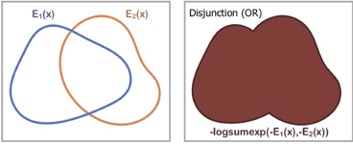### Negation

In concept negation, we wish to generate an output that does not contain the concept. Given a color red, we want an output that is of a different color, such as blue. Thus, we want to construct a distribution that places high likelihood to data that is outside a given concept. One choice is a distribution inversely proportional to the concept. Importantly, negation must be defined with respect to another concept to be useful. The opposite of alive may be dead, but not inanimate. Negation without a data distribution is not integrable and leads to a generation of chaotic textures which, while satisfying absence of a concept, is not desirable. Thus in our experiments with negation we combine it with another concept to ground the negation and obtain an integrable distribution: $p(x| \text{not}(c_1), c_2) \propto \frac{p(x|c_2)}{p(x|c_1)^\alpha} \propto e^{ \alpha E(x|c_1) - E(x|c_2) }$ Through our implicit sampling procedure, by assuming partition functions are equal, we can then generate samples using $\tilde{\mathbb{x}}^k = \tilde{\mathbb{x}}^{k-1} - \frac{\lambda}{2} \nabla_\mathbb{x} (\alpha E(x|c_1) - E(x|c_2)) + \omega^k$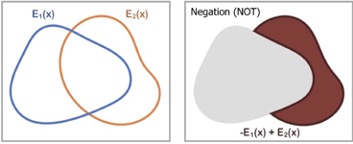By combining the above operators, we can controllably generate images with complex relationships. For example, given EBMs trained on male, smiling, and black haired faces, through combinations of negation, disjunction and conjunction, we can selectively generate images in a Venn diagram as shown below: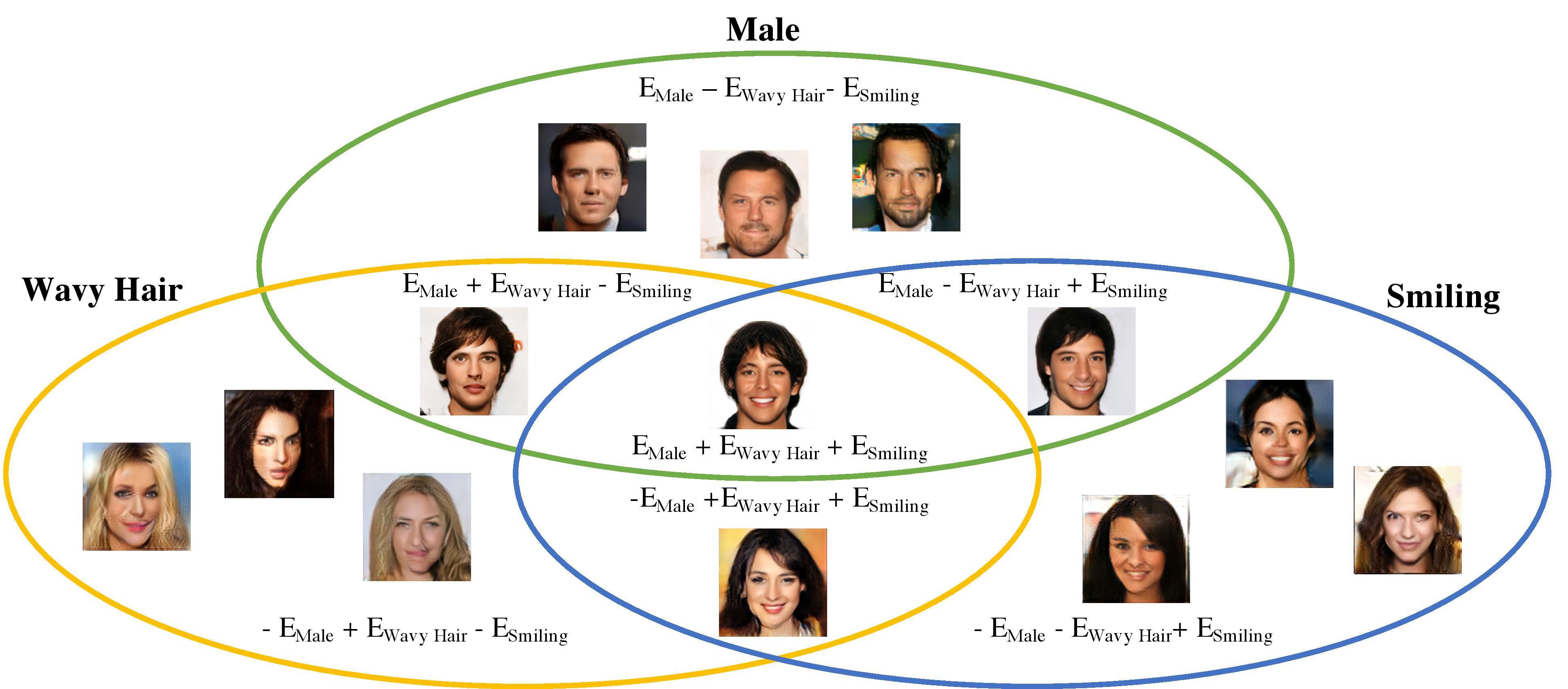## Compositional Generations

We first explore the ability of our models to compose across different attributes. We train seperate EBMs on the attributes of shape, position, size and color. Through conjunction on each model sequentially, we are able to generate successively more refined versions of an object scene.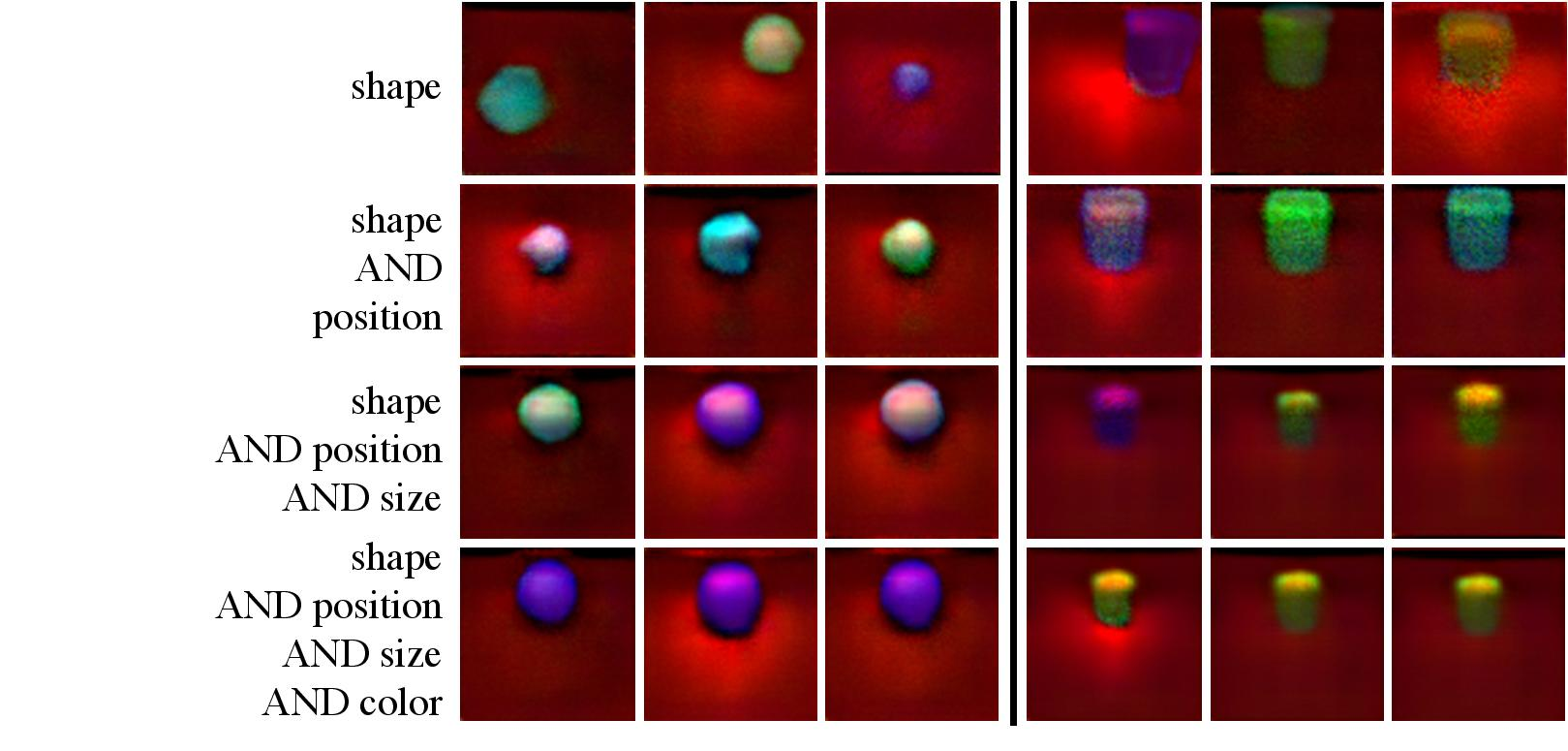We can similarily train seperate EBMs on the attributes of young, female, smiling, and wavy hair. Through conjunction on each model sequentially, we are able to generate successively more refined versions of human faces.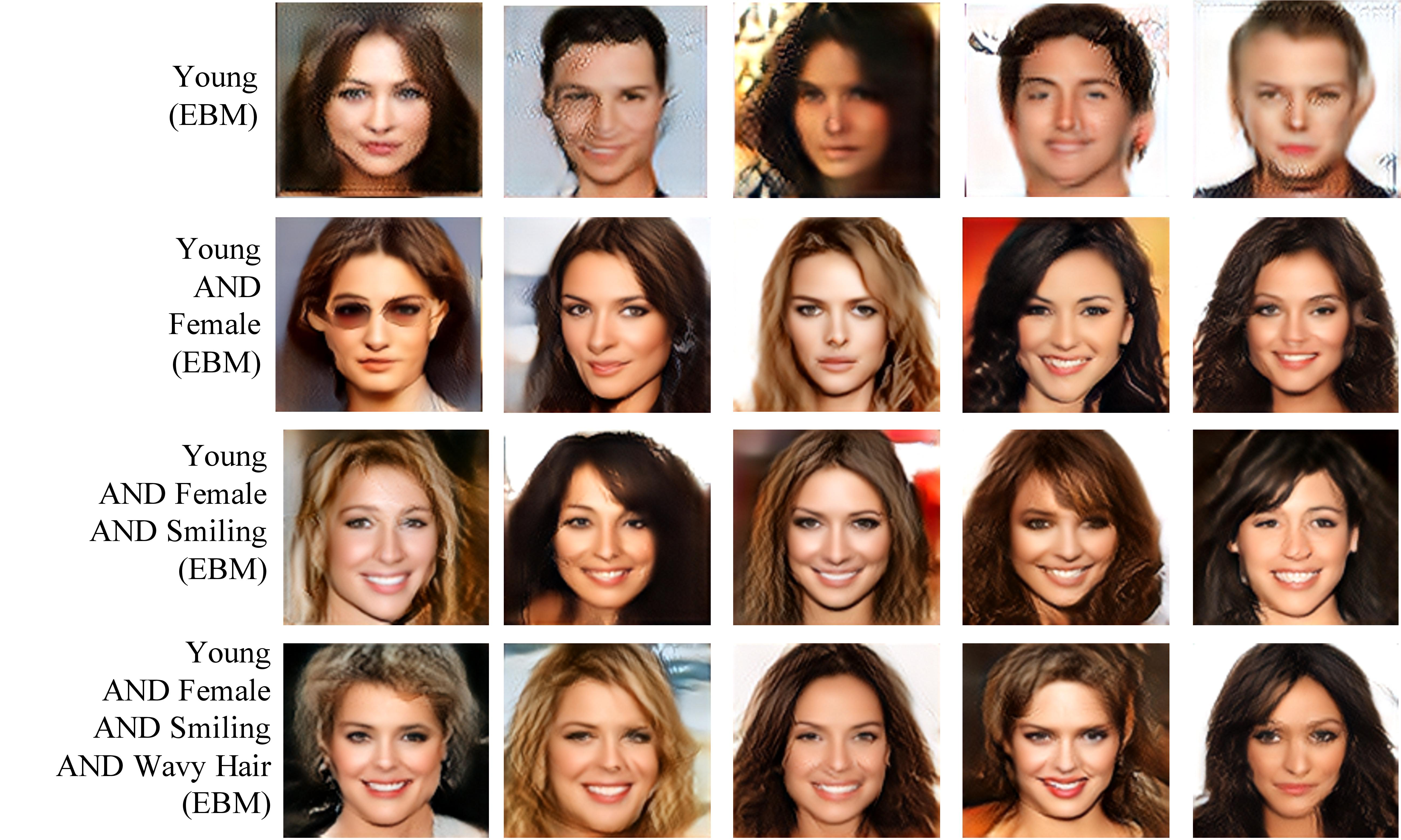Surprisingly, we find that generations of our model are able to become increasingly more refined by adding more models.

### Higher Level Compositions

We can further compose seperately trained models in additional ways by nesting operations of conjunction, disjunction and negation. In the figure below, we showcase face generations by nesting compositions of each operator.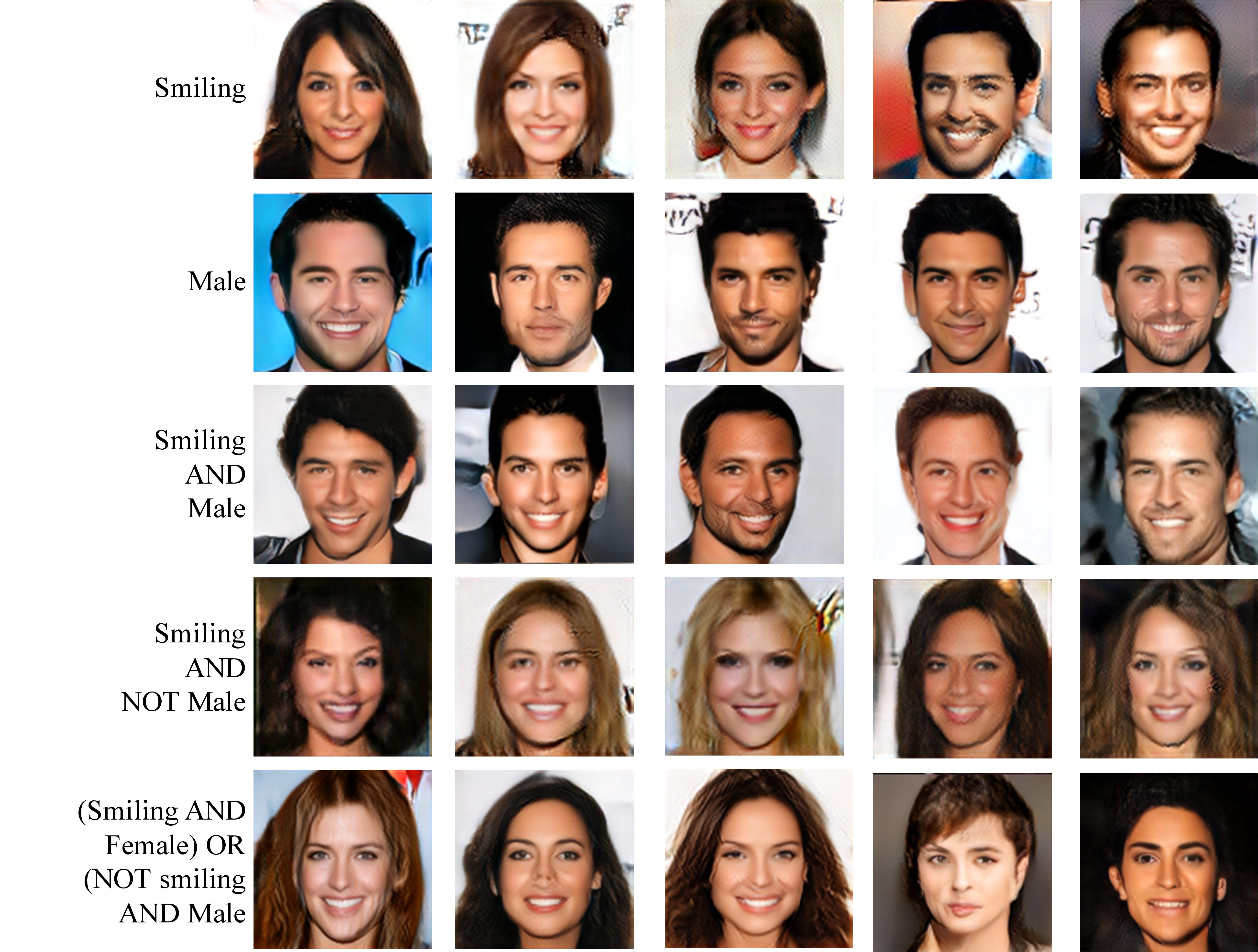### Object Level Compositionality

We can also learn EBMs on the object attributes. We train a single EBM model to represent the position attribute. By summing EBMs conditioned on two different positions (conjunction), we can compositionally generate different number of cubes at the object level.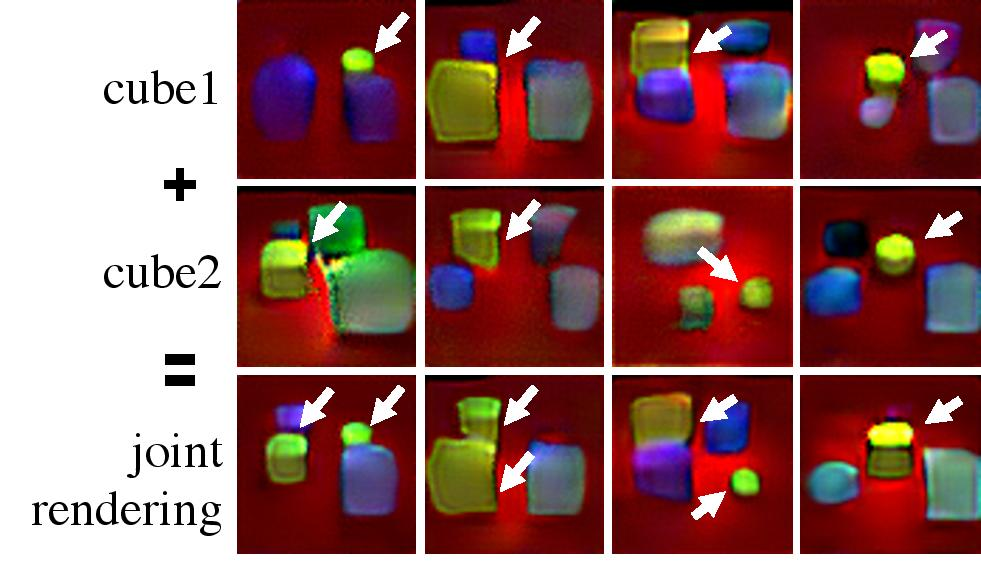Suprisingly, we find that when conditioned cubes are too close to each other, a single cube is instead genereated.

## Continual Learning in Generation

By having the ability to compose independently models, EBMs allow us to continually learn to generate new images with both new and old visual concepts. To test this we consider:

• A dataset consisting of position annotations of purple cubes at different positions.
• A dataset consisting of shape annotations of different purple shapes at different positions.
• A dataset consisting of color annotations of different color shapes at different positions.
We train a new EBM model for attributes of position, shape, and color, and find that composing our three attribute EBMs together, we can precisely generate shapes of different position, shape and color objects! This is inspite of the fact that the position and shape models have not seen many such possible combinations during training.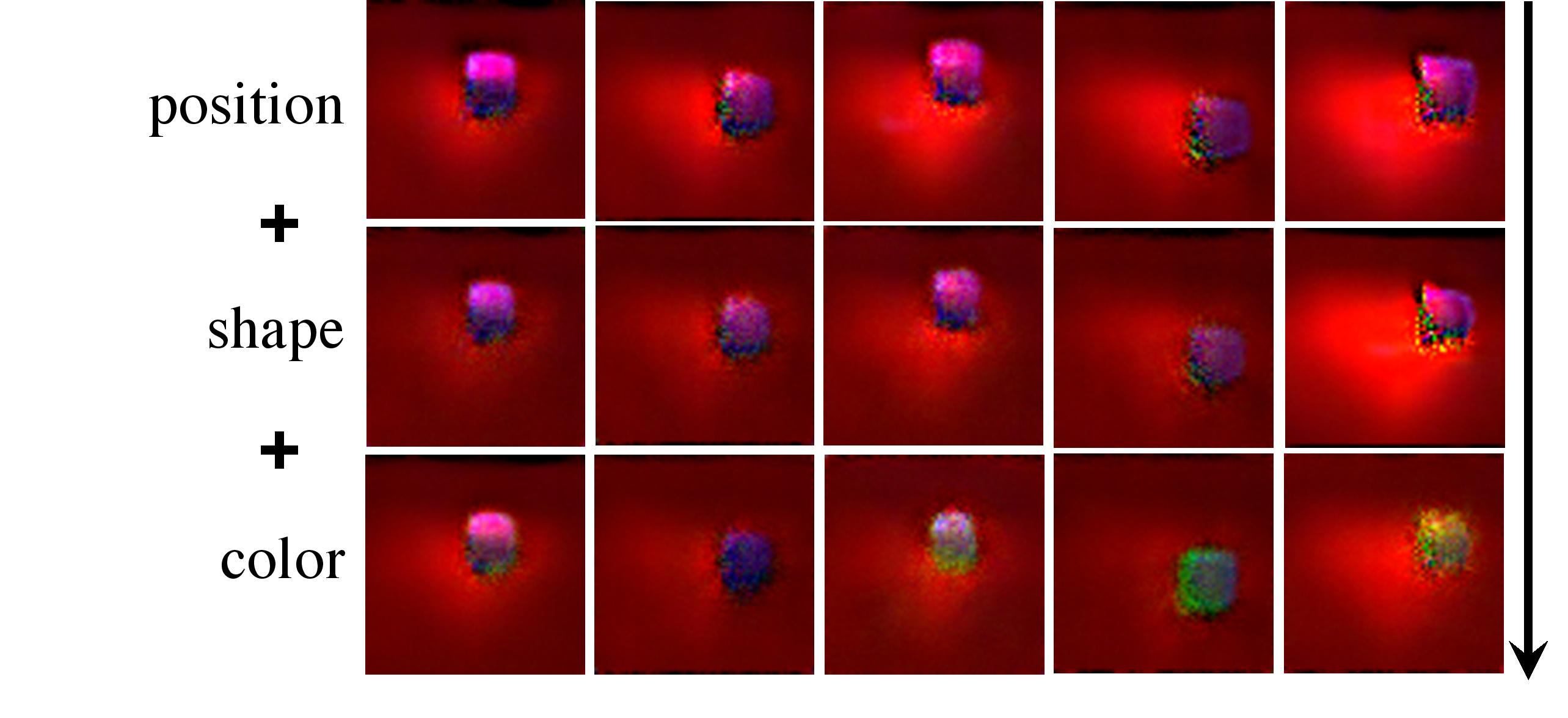## Compositional Inference

### Inference

In concept inference, we wish to infer the underlying concepts that best explains a given image. Given a learned EBM on $$E(x|c)$$, we do inference to find the underlying concept $$c$$ by $c = \text{argmin}_c E(x|c)$ If we know that a set of different $$x_i$$ that all have the same underlying concept $$c$$, we can then use conjunction to obtain , $c = \text{argmin}_c \sum_i E(x_i|c)$

### Compositional Inference Across Multiple Views

We test the above compositional reasoning on inferring the position of a cube given different view of the same image. By doing inference on the latent of EBM in the above manner, we find that we can obtain better predictions of positions.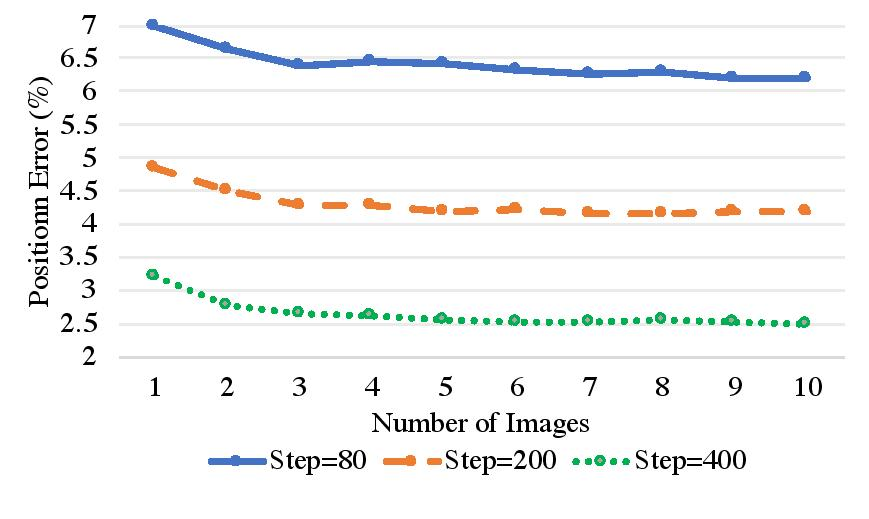### Compositional Inference In One Image

We can further test the ability of our model to implicitly compositionally infer the positions of multiple cubes when the model is trained on scenes with a single cube at different positions. We show the positions that are assigned low energy by our EBM in a heatmap below. We find that a single EBM can compositionally infer the presence of multiple cubes, despite only being trained on a single cube.